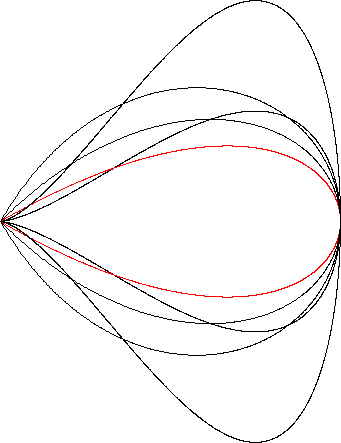Tschirnhausen Cubic
TSCHIRNHAUSEN CUBIC

Balmoral SoftwareThe lobe of the Tschirnhausen cubic is a convex x-symmetric droplet-shaped closed curve S with polar equation
r(t) = sec3(t/3), -π ≤ t < π
The curve follows a counterclockwise path as t increases, starting from the cusp (-8,0) at t = -π and proceeding through Quadrants III and IV to the right edge (1,0) at t = 0, then following a symmetric path through Quadrants I and II back to the cusp at t = π. The maximum ordinate of the Tschirnhausen cubic occurs at (-2,2) when t = 3π/4, so the width x height of its bounding rectangle is 9 x 4.

### Metrics

We have
r'(t) = tan(t/3)sec3(t/3),
so by (L2), the perimeter of the Tschirnhausen cubic is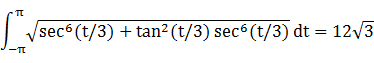and by (A2), its area A is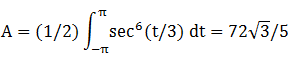By (C2), its centroid abscissa is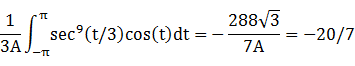### Incircle

The maximum radius of a circle centered on the x-axis and inscribed in S is its maximum ordinate, so a candidate for the incircle has radius R = 2 and center abscissa c = -2. The candidate circle must be contained within S, so we require that c - R and c + R both be within the abscissa range [-8,1] of S, which is true. For verification, we have### Inellipse

Using z = 1 in Lemma E,
d/dt [x(t) - z]y(t) = d/dt -3sin(t)tan2(t/3)sec3(t/3)
has a zero at t* = (3/2)Arccos(-2/7). The corresponding coordinates are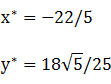We then have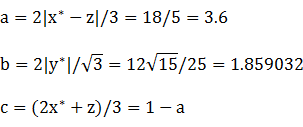For verification,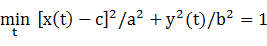### Circumellipse

Using z = -8 in Lemma E,
d/dt [x(t) - z]y(t) = d/dt [sec3(t/3)cos(t) + 8]sec3(t/3)sin(t)
has a zero at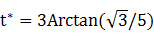The corresponding coordinates are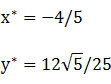We then haveFor verification,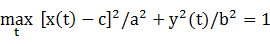### Circumcircle

Since the abscissa extrema are on the x-axis and the bounding rectangle is wider than it is tall, a candidate for the circumcircle has radius 9/2 and center abscissa -7/2. For verification, we have### Summary Table

 Perimeter Area Centroid Figure Parameters Incircle R = 2 12.566371 12.566371 (-2,0) Inellipse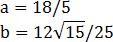17.588970 21.025157 (-2.6,0) Tschirnhausen cubic Width: 9Height: 4 20.784610 24.941532 (-2.857143,0) Circumellipse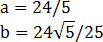22.627006 32.370342 (-3.2,0) Circumcircle R = 9/2 28.274334 63.617251 (-3.5,0)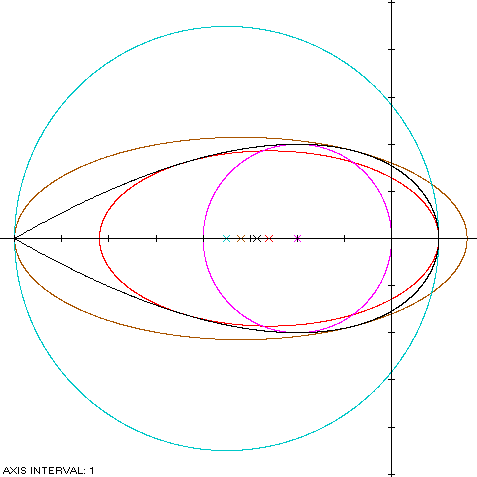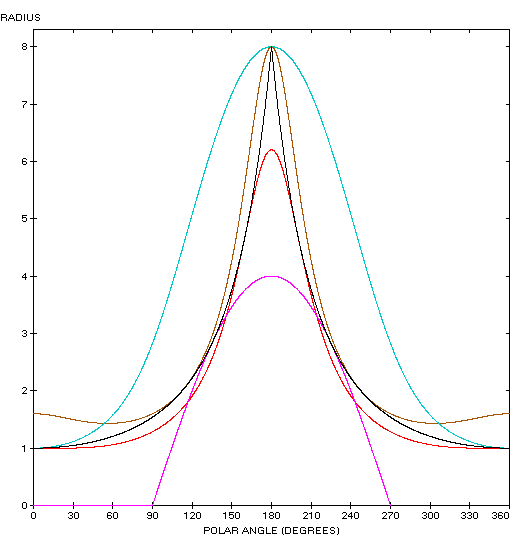The Tschirnhausen cubic (red) is a member of a group of similarly-shaped figures described on these pages, including (inside to outside) the teardrop curve, the right strophoid, the Trisectrix of Maclaurin and the piriform curve: